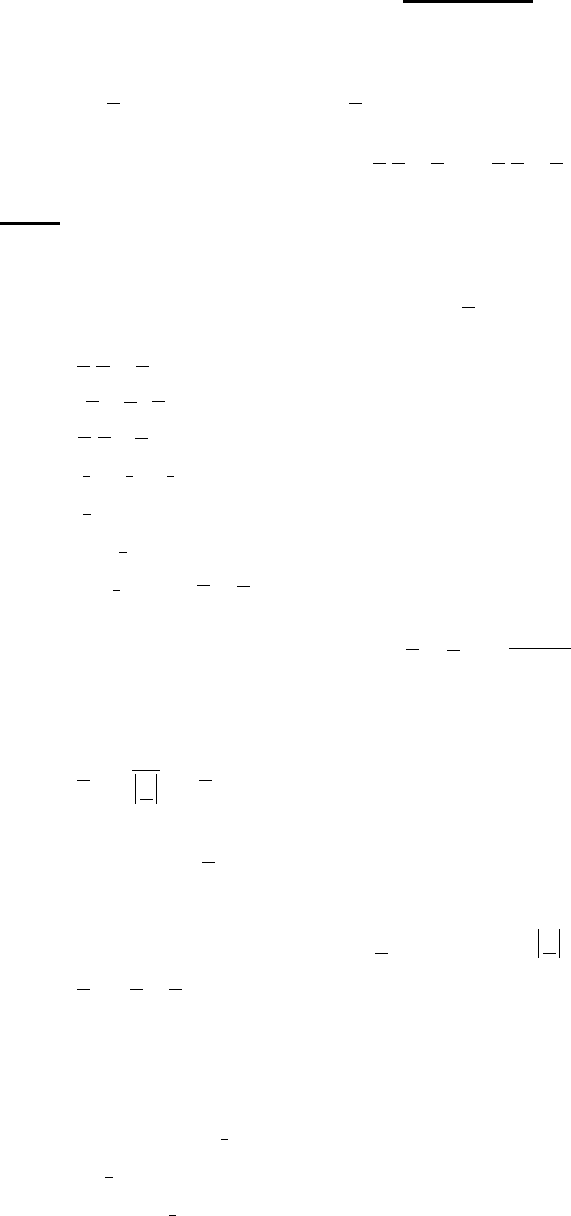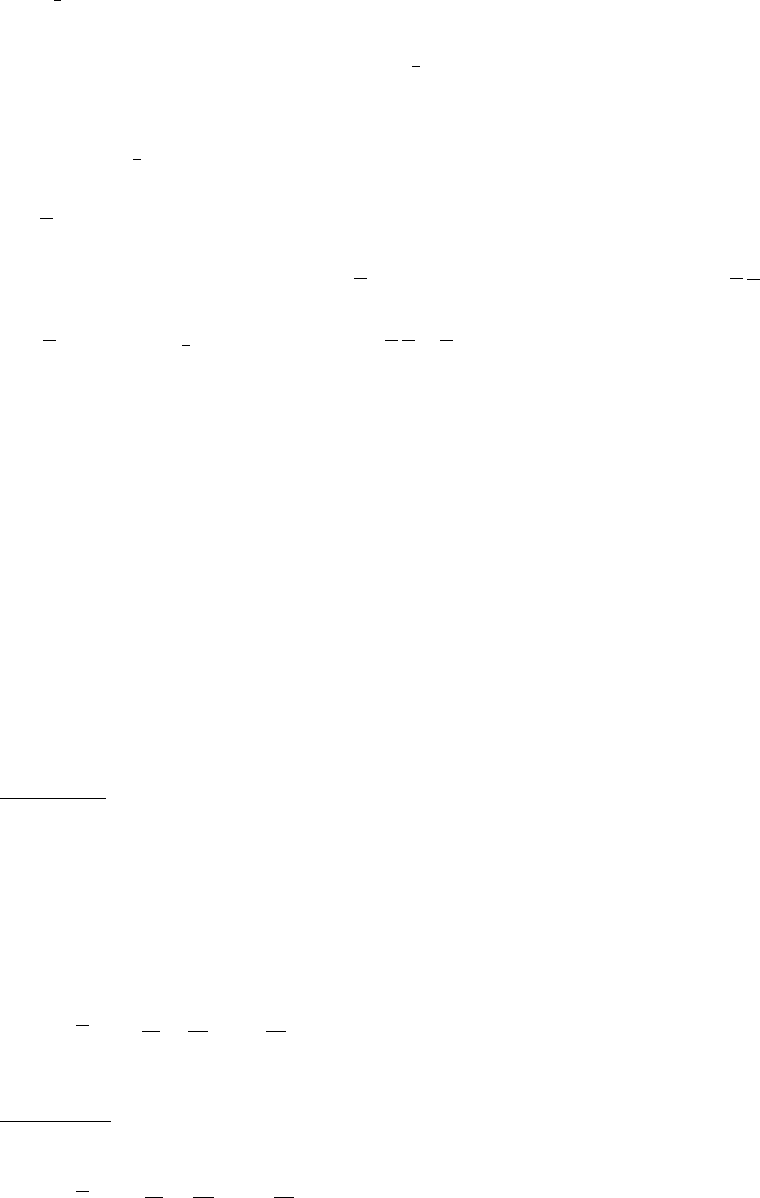Class Notes (1,100,000)
US (490,000)
UIC (1,000)
CHE (100)
CHE 311 (10)
Lecture 29

# CHE 311 Lecture Notes - Lecture 29: Surface Tension, Viscosity, List Of Forgotten Realms Nations

Department
Chemical Engineering
Course Code
CHE 311
Professor
Wedgewood Lewis
Lecture
29

This preview shows pages 1-2. to view the full 6 pages of the document.© 2017 Meenesh R. Singh (mrsingh@uic.edu)
Lecture 4
Inverse of
A
is defined as a matrix
B
such that
BA I
=
or
AB I
=
Prove. If inverse exists, it is unique.
If it is not unique, assume that there is a matrix
C
such that
CA I=
( )
0B CA−=
0
DA=
0
,
DA
rrrn+−
,
A
rn=
0
D
r
00
D
rD=→=
Q.E.D
We know that,
1
A
=
Suppose the rank of
A
is
1n
A
is singular
0A→=
Apply Sylvester’s Law of Nullity,
( )
01
n rn≥ −+
1
r
01
r→≤ ≤

Only pages 1-2 are available for preview. Some parts have been intentionally blurred.© 2017 Meenesh R. Singh (mrsingh@uic.edu)
if
0
r= →
Every cofactor is zero but
10
A
rn= −≠
.
At least one cofactor is non-zero
1
r→=
has at least one non-zero column vector if there are more than one column of non-zero
vector, they must be linearly dependent.
Any non-zero column of
is a solution to the homogenous eq:
0Ax=
.
Let
A
has a rank
A
r
. We want to solve
0Ax=
.
11 1 12 2 1 1, 1 1 1 1
0
rr r r n n
ax ax ax a x ax
++
+ +…+ + +…+ =
21 1 22 2 2 2, 1 1 2 0
rr r r nn
ax ax ax a x ax
++
+ +…+ + +…+ =
11 2 2 , 1 1 0
r r rr r r r r rn n
ax ax ax a x ax
++
+ +…+ + +…+ =
11 2 2 , 1 1
0
n n nr r n r r nn n
ax ax ax a x ax
++
+ +…+ + +…+ =
we are solving for upper left hand corner.
12
,,,
r
xx x
are unknowns.
12
, ,,
rr n
xx x
++
can be assumed.
1st solution
Assume
1
1
r
x
+
=
0, 2, ,
j
x jr n= =+…
Solve,
()() () ( )
11 1
112
, , , ,1 , 0, , 0 T
r
x xx x

= ……

2nd solution
( ) ( ) ( ) ( )
22 2
212
, , , , 0,1 , 0, , 0
T
r
x xx x

= ……
